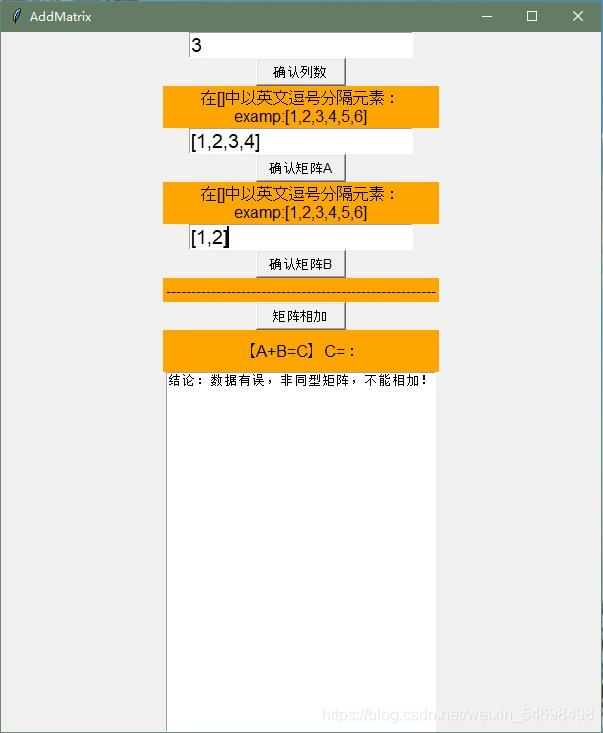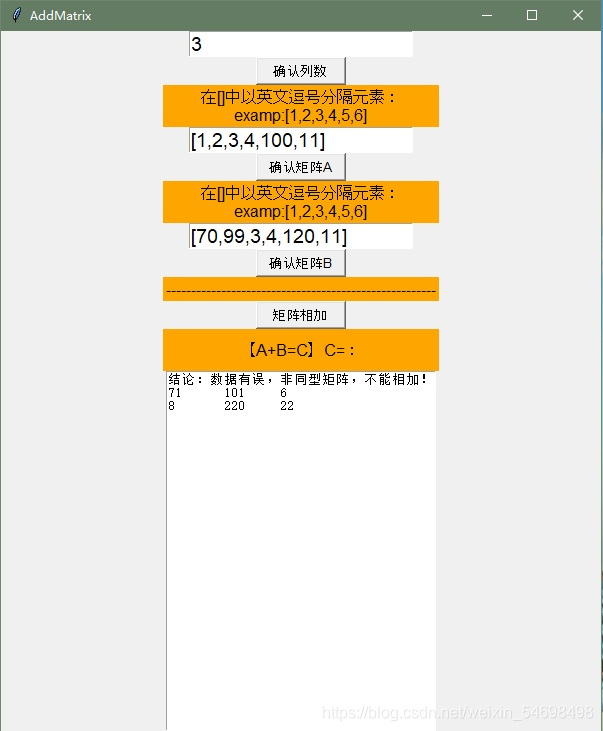• python矩阵相加
2020-12-15 22:49:50

【摘要】今天的python实践内容是为了让大家了解python矩阵相加方法，对代码编程有个感性的认知。也好让大家能够理性选择，不要盲目跟从，选择适合自己当前阶段的学习内容，循序渐进，以兴趣自我探索为向导，所以这次的python矩阵相加方法你需要了解。

python矩阵相加题目：

两个 3 行 3 列的矩阵，实现其对应位置的数据相加，并返回一个新矩阵：

X = [[10,7,8],

[4 ,5,6],

[7 ,2,9]]

Y = [[5,7,1],

[6,7,3],

[4,6,9]]

python矩阵相加程序分析：

创建一个新的 3 行 3 列的矩阵，使用 for 迭代并取出 X 和 Y 矩阵中对应位置的值，相加后放到新矩阵的对应位置中。

程序源代码:

#!/usr/bin/python

# -*- coding: UTF-8 -*-

X = [[10,7,8],

[4 ,5,6],

[7 ,2,9]]

Y = [[5,7,1],

[6,7,3],

[4,6,9]]

result = [[0,0,0],

[0,0,0],

[0,0,0]]

# 迭代输出行

for i in range(len(X)):

# 迭代输出列

for j in range(len(X)):

result[i][j] = X[i][j] + Y[i][j]

for r in result:

print(r)

这次的python矩阵相加方法就为大家介绍到这里了，python作为一款实用性极强的编程软件，可以为大家做很多事情，当然前提是你对编程有一颗热爱的心，如果你想做好python，就试试将他用在实践里，如果想了解更多python教程，可以点击下方资料下载链接。

更多相关内容
• python 矩阵相加 正整数n，代表要输入的是n*n的矩阵，两个矩阵由用户输入，计算输出两个矩阵相加的和。

## 矩阵相加

描述

正整数n，代表要输入的是n*n的矩阵，两个矩阵由用户输入，计算输出两个矩阵相加的和。

输入
一个整数n，和两个矩阵的值。

输出
两个矩阵相加后的值，每个元素占三位，中间对齐，元素之间由两个空格组成。

输入样例 1

3
12 3 -5
1 -9 0
3 6 8
1 8 9
6 5 4
3 2 1
输出样例 1

13 11 4
7 -4 4
6 8 9

n = int(input())
x = []
for i in range(n):
x.append(list(map(int, input().rstrip().split())))#用户输入第一个矩阵
y = []
for j in range(n):
y.append(list(map(int, input().rstrip().split())))#用户输入第二个矩阵
result=[[0 for i in range(n)]for j in range(n)]  #建立一个用来放计算结果的空矩阵
for i in range(len(x)):
for j in range(len(x[i])):
result[i][j]=x[i][j]+y[i][j] #将相加的结果放入到空矩阵中
for i in range(n):#矩阵的输出
for j in range(n):
print(f'{result[i][j]:^3}',end='  ')
print()

展开全文### Python——矩阵相加（采用矩阵相加数学计算方法实现）（tkinter实现）

[功能模块作者]
CSDN用户（TDTX），TDTX主页——负责矩阵相加功能

【矩阵相加】采用线性代数中，计算矩阵相加的方法实现
1.在点击“矩阵相加”之前，要先点击其余所有按钮
2.本文具有能否相加的输入检查
3.矩阵的输入方式：将每行依次输入，变成行数为1的矩阵输入

如：
4 5 6
1 2 3 输入为：[4,5,6,1,2,3]

def addmatrix():
import tkinter as tk
linearwindow1=tk.Tk()
linearwindow1.geometry("600x700")
def lie():
lie=eval(e1.get())
def smatrix1():
global ma1
ma1=eval(e2.get())
def smatrix2():
global ma2
ma2=eval(e3.get())
lie=eval(e1.get())

#        print(ma1)
#        print(ma2)
le=len(ma1)
#        print(le)
co=0
i=0
if len(ma1)==len(ma2) and len(ma1)>0 and len(ma2)>0 and (len(ma1)%lie)==(len(ma2)%lie) and len(ma1)%lie==0 and len(ma2)%lie==0:
while i<=le-1:
i=i+1
i=0
while i<=le-1:
co=co+1
if co%lie!=0:
tx.insert('insert','\t')
if co%lie==0:
tx.insert('insert','\n')
i=i+1
tx.insert('insert','\n')
else:
tx.insert('insert',"结论：数据有误，非同型矩阵，不能相加！")
e1 = tk.Entry(linearwindow1,font=('Arial', 14))
e1.pack()
bt1=tk.Button(linearwindow1,text='确认列数',width=10,height=1,font=('Arial', 10),command=lie)
bt1.pack()

lb1=tk.Label(linearwindow1, text='在[]中以英文逗号分隔元素：\nexamp:[1,2,3,4,5,6]', bg='orange', font=('Arial', 12), width=30, height=2)
lb1.pack()
e2 = tk.Entry(linearwindow1,font=('Arial', 14))
e2.pack()
bt2=tk.Button(linearwindow1,text='确认矩阵A',width=10,height=1,font=('Arial', 10),command=smatrix1)
bt2.pack()

lb2=tk.Label(linearwindow1,text='在[]中以英文逗号分隔元素：\nexamp:[1,2,3,4,5,6]', bg='orange', font=('Arial', 12), width=30, height=2)
lb2.pack()
e3 = tk.Entry(linearwindow1,font=('Arial', 14))
e3.pack()
bt3=tk.Button(linearwindow1,text='确认矩阵B',width=10,height=1,font=('Arial', 10),command=smatrix2)
bt3.pack()

lb3=tk.Label(linearwindow1,text='------------------------------------------------------', bg='orange', font=('Arial', 12), width=30, height=1)
lb3.pack()
bt4.pack()
lb4=tk.Label(linearwindow1,text='【A+B=C】C=：', bg='orange', font=('Arial', 12), width=30, height=2)
lb4.pack()

tx=tk.Text(linearwindow1,width=38,height=30)
tx.pack()
linearwindow1.mainloop()


# 2.结果示例

【若不符合A+B的运算条件，则会输出结论提示】【符合运算条件，输出结果】展开全文tkinter
• 【摘要】今天的python实践内容是为了让大家了解python矩阵相加方法，对代码编程有个感性的认知。也好让大家能够理性选择，不要盲目跟从，选择适合自己当前阶段的学习内容，循序渐进，以兴趣自我探索为向导，所以这次...

【摘要】今天的python实践内容是为了让大家了解python矩阵相加方法，对代码编程有个感性的认知。也好让大家能够理性选择，不要盲目跟从，选择适合自己当前阶段的学习内容，循序渐进，以兴趣自我探索为向导，所以这次的python矩阵相加方法你需要了解。

python矩阵相加题目：

两个 3 行 3 列的矩阵，实现其对应位置的数据相加，并返回一个新矩阵：

X = [[10,7,8],

[4 ,5,6],

[7 ,2,9]]

Y = [[5,7,1],

[6,7,3],

[4,6,9]]

python矩阵相加程序分析：

创建一个新的 3 行 3 列的矩阵，使用 for 迭代并取出 X 和 Y 矩阵中对应位置的值，相加后放到新矩阵的对应位置中。

程序源代码:

#!/usr/bin/python

# -*- coding: UTF-8 -*-

X = [[10,7,8],

[4 ,5,6],

[7 ,2,9]]

Y = [[5,7,1],

[6,7,3],

[4,6,9]]

result = [[0,0,0],

[0,0,0],

[0,0,0]]

# 迭代输出行

for i in range(len(X)):

# 迭代输出列

for j in range(len(X)):

result[i][j] = X[i][j] + Y[i][j]

for r in result:

print(r)

这次的python矩阵相加方法就为大家介绍到这里了，python作为一款实用性极强的编程软件，可以为大家做很多事情，当然前提是你对编程有一颗热爱的心，如果你想做好python，就试试将他用在实践里，如果想了解更多python教程，可以点击下方资料下载链接。

展开全文• python对于矩阵的运算。简单的方法，快速实现。并可延申至多维矩阵的计算
• **题目：**计算两个矩阵相加。 **程序分析：**创建一个新的矩阵，使用 for 迭代并取出 X 和 Y 矩阵中对应位置的值，相加后放到新矩阵的对应位置中。 X = [[12,7,3], [4 ,5,6], [7 ,8,9]] Y = [[5,8,1], [6,7,...编程练习
• # 题目：计算两个矩阵相加。 # 程序分析：创建一个新的矩阵，使用 for 迭代并取出 X 和 Y 矩阵中对应位置的值，相加后放到新矩阵的对应位置中。
• 参考链接： Python中的sum函数 假如矩阵A是n*n的矩阵 A.sum（）是计算矩阵A的每一个元素之和。...A.sum(axis=0)是计算矩阵每一列元素相加之和。 A.Sum(axis=1)是计算矩阵的每一行元素相加之和。
• ## python矩阵相加

千次阅读 2019-03-06 20:09:00
# 使用 for 迭代并取出 X 和 Y 矩阵中对应位置的值，相加后放到新矩阵的对应位置中。 import numpy as np a = np.random.randint(1,100,(3,3)) print(a) b = np.random.randint(1,100,(3,3)) p...
• 矩阵 python 加法Here you will get program for python matrix addition. In python “list” concept is there rather than arrays. For implementing matrix we should take help of lists in python. Matrix can...列表 算法 机器学习 深度学习
• def matrix_add(matrix1,matrix2): total_element = [] new_matrix = [] total_element = [matrix1[i][j] + matrix2[i][j] for i in range(len(matrix1)) for j in range(len(matrix1))] new_matr...
• 今天小编就为大家分享一篇对python矩阵相加函数sum()的使用详解，具有很好的参考价值，希望对大家有所帮助。一起跟随小编过来看看吧
• 假如矩阵A是n*n的矩阵A.sum（）是计算矩阵A的每一个元素之和。A.sum(axis=0)是计算矩阵每一列元素相加之和。A.Sum(axis=1)是计算矩阵的每一行元素相加之和。...numpy sum
• 两个 3 行 3 列的矩阵，实现其对应位置的数据相加，并返回一个新矩阵： X = [[12,7,3], [4 ,5,6], [7 ,8,9]] Y = [[5,8,1], [6,7,3], [4,5,9]] 分析 可以按照数学计算的过程，让对应数字相加。当然如果使用numpy库那...
• 数据是一个二维矩阵，但是在这里你可以用一个索引来访问它。data[k]因此是一整行，使用+可以连接行(可能不是您想要的，对吗？)。可能highBandWidth的解决方案正是您所寻找的。在第二个问题更微妙，是关于陈述^{pr2}\$...
• 本文实例讲述了python实现矩阵加法和乘法的方法。分享给大家供大家参考，具体如下：本来以为python矩阵用list表示出来应该很简单可以搞。。其实发现有大学问。这里贴出我写的特别不pythonic的矩阵加法，作为反例。...
• 使用python和R进行科学计算，最为人所诟病的是它们的运算速度。然而，通过关联Intel MKL线性代数库，你可以使python和R达到和matlab类似的运算速度，并且自动使用闲置CPU核心进行并行。r语言
• ## python矩阵_Python矩阵

千次阅读 2020-07-14 04:34:52
python矩阵In this tutorial we will learn about Python Matrix. In our previous tutorial we learnt about Python JSON operations. 在本教程中，我们将学习Python矩阵。 在上一教程中，我们了解了Python JSON...numpy 机器学习 人工智能 tensorflow
• 主要介绍了Python实现的矩阵转置与矩阵相乘运算,结合实例形式分析了Python针对矩阵进行转置与相乘运算的相关实现技巧与操作注意事项,需要的朋友可以参考下
• # =============================================================================python矩阵运算分为两种形式，一是np.array，而是np.matrix# ==============================================================...
• 本文转自：http://blog.sina.com.cn/s/blog_5f234d4701012p64.html科学计算：Python VS....本文主要设涉及线性代数和矩阵论的基本内容。先回顾这部分理论基础，然后给出MATLAB，继而给出Python的处理。个人...
• python下：列表!=数组!=矩阵 # python列表 [1,2]+[3,4]=[1,2,3,4] # np数组 np.array([1,2])+np.array([1,2])=array([2, 4]) # tensor矩阵 torch.tensor([1,2])+torch.tensor([1,2])=tensor([2, 4]) # broadcast a =...pytorch
• #矩阵加法、减法和数乘 print("Choose a function: 'addition', 'subtraction' or 'multiplication': ") function = input() #选择功能 if function == "addition" or function == "subtraction": matrix_1 = [] #...
• 今天小编就为大家分享一篇python中利用numpy.array()实现俩个数值列表的对应相加方法，具有很好的参考价值，希望对大家有所帮助。一起跟随小编过来看看吧...